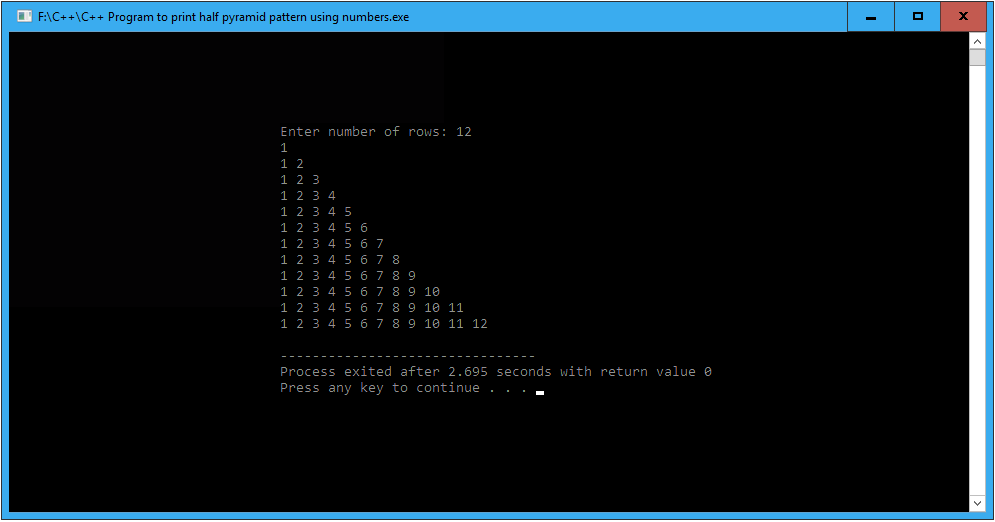# C++ Program to print half pyramid pattern using numbers

In this article write a C++ Program to print half pyramid pattern using numbers. This Program first takes the numbers of rows and uses nested for loops to print half pyramid pattern.

## C++ Program to print half pyramid pattern using numbers

```//C++ Program to print half pyramid pattern using numbers

#include <iostream>
using namespace std;

int main()
{
int rows, i, j;

cout << "Enter number of rows: ";
cin >> rows;

// outer loop is responsible for row
for(i = 1; i <= rows; i++)
{
//inner loop is responsible for columns
for(j = 1; j <= i; j++)
{
cout << j << " ";
}
// give line breaks after ending every row
cout << "\n";
}
return 0;
}
```

### When the above C++ program is compile and run, it will produce the following result: